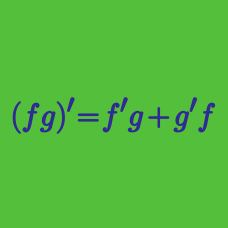Calculus

# Applying Multiple Differentiation Rules

If $f(x) = \ln \left(\frac{x + 7}{\sqrt{x-2}}\right)$, $f'(18)$ can be expressed as $\frac{a}{b}$, where $a$ and $b$ are coprime positive integers. What is the value of $a+b$?

The derivative of $y=(x-9)^3(x^2+6)^2$ can be expressed as $\frac{dy}{dx}=(x-9)^2(x^2+6) \cdot f(x).$ What is $f(x)?$

If $\displaystyle y=\sin^{-1}(x^{5}-2),$ what is $\displaystyle \frac{dy}{dx}?$

If the derivative of $\displaystyle f(x)=\left(\frac{x+7}{6x+1}\right)^{5}$ is expressed as $\displaystyle \frac{-a(x+7)^b}{(6x+1)^{6}}$ for integers $a$ and $b$, what is $a+b?$

If $\displaystyle y=4x\tan^{-1}\sqrt{x},$ what is $\displaystyle \frac{dy}{dx}?$

×